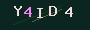# array_multisort二维或多维数组或多个数组的排序实例详解

php的array_multisort() 函数对多个数组或多维数组进行排序，字符串键名（key）将被保留，但是数字键名将被重新索引，从 0 开始，并以 1 递增。

### 1、字符串索引和数字索引

`\$a=array('b','a','c'); \$b=array('a'=>1,'b'=>3,'c'=>2); array_multisort(\$a); /* \$a=Array (  => a  => b  => c ) \$b=Array ( [a] => 1 [c] => 2 [b] => 3 ) */`

### 2、两个数组排序

`\$a=array('b','a','c'); \$b=array('a'=>1,'b'=>3,'c'=>2); array_multisort(\$a,\$b); /* \$a=Array (  =>&n`

### 有 1 位网友评论：array_multisort这个函数不好理解，感谢博主，跟着一步一步看，终于摸透了这个函数~~~^︶^~~~

### 欢迎 你 来评论• QQ
• 二维码支付宝赞助打赏微信赞助打赏